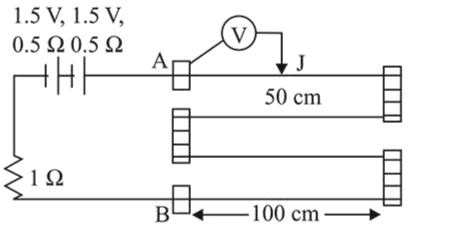# In the circuit shown, a four-wire potentiometer is made

Question:

In the circuit shown, a four-wire potentiometer is made of a $400 \mathrm{~cm}$ long wire, which extends between $\mathrm{A}$ and $\mathrm{B}$. The resistance per unit length of the potentiometer wire is

$r=0.01 \Omega / \mathrm{cm}$. If an ideal voltmeter is connected as shown with jockey $\mathrm{J}$ at $50 \mathrm{~cm}$ from end $\mathrm{A}$, the expected reading of the voltmeter will be :1. (1) $0.50 \mathrm{~V}$

2. (2) $0.75 \mathrm{~V}$

3. (3) $0.25 \mathrm{~V}$

4. (4) $0.20 \mathrm{~V}$

Correct Option: , 3

Solution:

(3) The resistance of potentiometer wire

$\mathrm{R}=0.01 \times 400=4 \Omega$

Current in the wire

$i=\frac{V}{R_{T}}=\frac{3}{4+0.5+0.57+1}=\frac{1}{2} A$

Now $V=i R_{\mathrm{AJ}}=\frac{1}{2} \times(0.01 \times 50)=0.25 \mathrm{~V}$